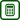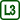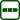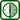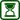## Measuring Refractive Index Using Digital Rangefinder

### Experiment number : 2262

• #### Goal of Experiment

The aim of this experiment is to determine the refractive index of various liquids using a digital rangefinder.

• #### Theory

The digital rangefinder is used for non-contact determination of the distance of a given object. The device emits a laser beam, which is reflected from the measured object, and the rangefinder sensor records the return of the reflected beam and the time elapsed between its sending and receiving. From this data, the rangefinder evaluates the distance of the object as the product of the speed of light in the air and half the time between sending and receiving the beam.

In its calculation, the rangefinder takes into account the speed of light in air (for simplicity, we will identify it in the following description with the speed of light in vacuum c). If we place another substance in the path of the beam, in which the light moves more slowly, the rangefinder will evaluate the distance inaccurately – as greater than what it actually is. We will use this “inaccuracy” in this experiment. If we denote as l1 the distance that the beam actually travels in the transparent substance, and l2 the distance that the rangefinder measures, we obtain the refractive index of the substance n as

$n=\frac{l_\mathrm{2}}{l_\mathrm{1}}.\tag{1}$
• #### Equipment

• digital rangefinder (see Fig. 1)
• transparent container (we used glass in the sample experiment)
• transparent substances with refractive index we want to measure
• reflective opaque surface (box, wall, blackboard ...)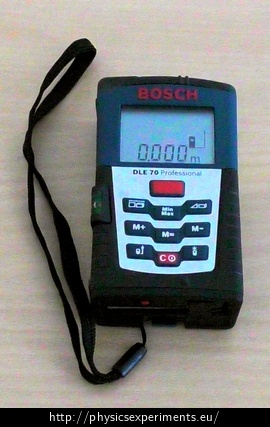• #### Procedure – measuring while neglecting the container walls

We will now describe a measurement that does not consider the effect of the walls of the container filled with the studied liquid. More specifically, its results correspond to a situation where the refractive index of the container walls would be the same as the refractive index of the liquid.

1. We place a reflective surface (a paper box) against one wall of the glass container and a digital rangefinder against the opposite wall.

2. We remove the container carefully so that the rangefinder or the reflecting surface do not move.

3. We measure the distance l1 with the rangefinder (Fig. 2 on the left).

4. Then we place the container back, pour the liquid into it and measure the distance l2 with the rangefinder (Fig. 2 on the right).

• #### Sample Result

We measured the refractive index of water and technical alcohol in a glass aquarium. The refractive index of the liquid is obtained according to formula (1), the measured and calculated values are shown in Table 1. In the last row of the table there are theoretically given values of the refractive index.

Table 1  substance water alcohol l1 [m] 0.449 0.449 l2 [m] 0.588 0.601 ncalculated 1.31 1.34 ntheoretical 1.33 1.36

It can be seen that the refractive index values obtained for both liquids agree very well with the theoretical values.

• #### Technical Notes

• We can simplify the calculation by neglecting the wall of the container only if these walls are thin compared to the path travelled by the light in the liquid. If we do not want to neglect the influence of the walls, the calculation of the refractive index is more complicated and it is described in the section Measuring Refractive Index Using Digital Rangefinder, More accurate procedure – measurement without neglecting the walls of the container.

• With some liquids (e.g. liquids with higher viscosity), the beam may be scattered in such a way that the rangefinder will not detect the reflected beam.

• Measurements can be performed not only for liquids but also for transparent solids.

• Measuring the refractive index of gases does not make reasonable sense, as these refractive indices are under normal conditions very close to one.

• #### Pedagogical Notes

• Whether or not we include the influence of the container walls on the measurement in its numerical evaluation, it is appropriate to draw students’ attention to the fact that the walls actually affect the measurement.

• If we explain to students how a digital rangefinder works, they can try to figure out themselves how to use it to measure the refractive index of the liquid.

• #### More accurate procedure – measurement without neglecting the walls of the container

If we want to include the effect of the container in the measurement and subsequent calculation, we will perform points 1 to 4 in the Procedure section in the same way, but we will also add another measurement – use the calliper to determine the thickness d of the container wall (Fig. 3).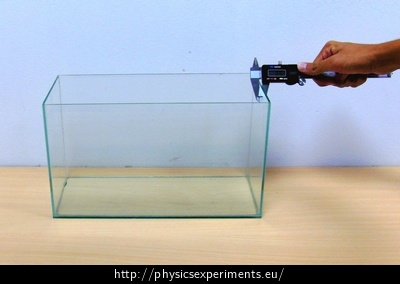We will now calculate the refractive index n of the liquid. The rangefinder always considers the measured environment to be air, i.e. when measuring the path l2 the time the beam travelled from the rangefinder to the reflecting surface is equal to

$t=\frac{l_2}{c}.$

In reality, however, we know that the beam had to pass through two glass walls of a container with a total width of 2d during this time and of course also through the liquid-filled space between them of length $$l_1-2d$$. Therefore, the following applies:

$t=\frac{2d}{\frac{c}{n_\mathrm{g}}}+\frac{l_1-2d}{\frac{c}{n}},$

where ng is the refractive index of glass and n is the refractive index of the liquid we want to determine. Both equations are true for the same time t. From this equality we determine the unknown refractive index n:

$n=\frac{l_2-2dn_\mathrm{g}}{l_1-2d}.$

The calculation assumes that we know the refractive index of the glass (in general: the material of the container), or we can derive it in a similar way as we have just done for the refractive index of the liquid. Substituting our values (refractive index of glass ng ≈ 1.5 and d = 3 mm), we obtain a refractive index of 1.31 for water and 1.34 for alcohol – the same values we got when we didn’t take the container walls into account. It is thus clear that the effect of the walls of the container is really insignificant at its sufficient length.

• #### Comment

The sensor recording the reflected beam in the rangefinder works on the principle of photoresistance. The reflected beam penetrates the sensor and changes the resistance of the component. It is then reflected in the change of electric current. This is a signal for the electronics in the rangefinder to evaluate the distance of the object from the measured time and constant c.